>科技>正文

# 【算法】从头开始编写任何机器学习算法的6个步骤：感知器案例研究

1 您如何学习机器学习算法？

2 您如何应用机器学习算法？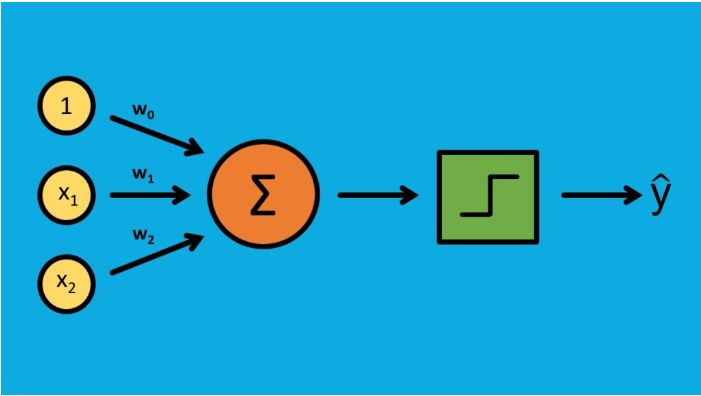1. 对算法有一个基本的了解
2. 找到一些不同的学习来源
3. 将算法分解成块
4. 从一个简单的例子开始
5. 使用可信的实现进行验证
6. 写下你的过程

• 它是什么?
• 它的典型用途是什么?
• 我什么时候不能用这个?

• 单层感知器是最基本的神经网络。它通常用于二进制分类问题(1或0，“是”或“否”)。
• 一些简单的用法可能是情感分析(正面或负面反应)或贷款违约预测(“将违约”，“将不违约”)。对于这两种情况，决策边界都必须是线性的。
• 如果决策边界是非线性的，你就不能用感知器。对于这些问题，您需要使用不同的方法。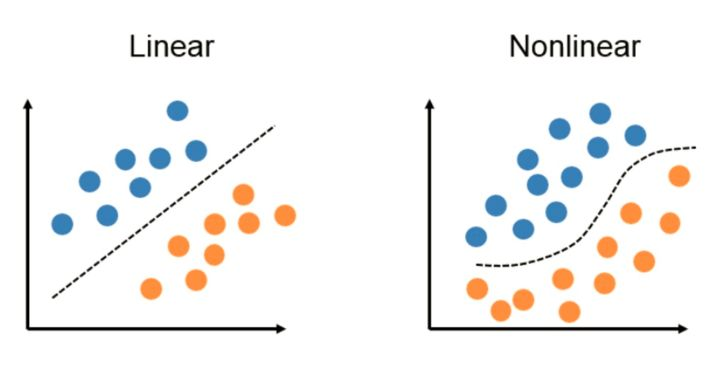• The Elements of Statistical Learning 4.5.1节
• Understanding Machine Learning: From Theory To Algorithms 21.4 节

• How To Implement The Perceptron Algorithm From Scratch In Python, by Jason Brownlee
• Single-Layer Neural Networks and Gradient Descent, by Sebastian Raschka

• Perceptron Training How the Perceptron Algorithm Works

• 初始化权重
• 将权重乘以输入，然后求和
• 将结果与阈值进行比较以计算输出(1或0)
• 更新权重
• 重复

1 初始化权重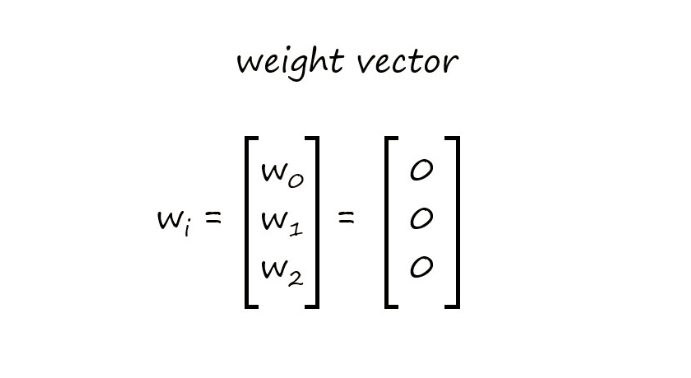2 将权重乘以输入，然后求和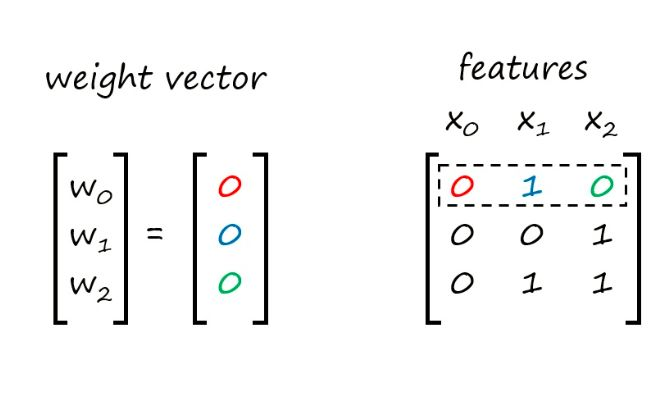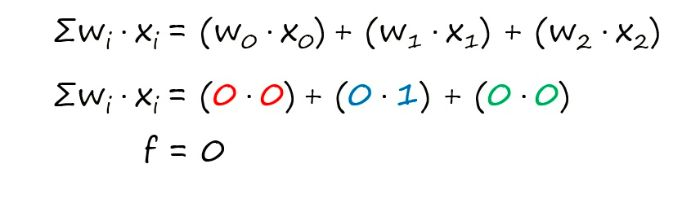3 比较阈值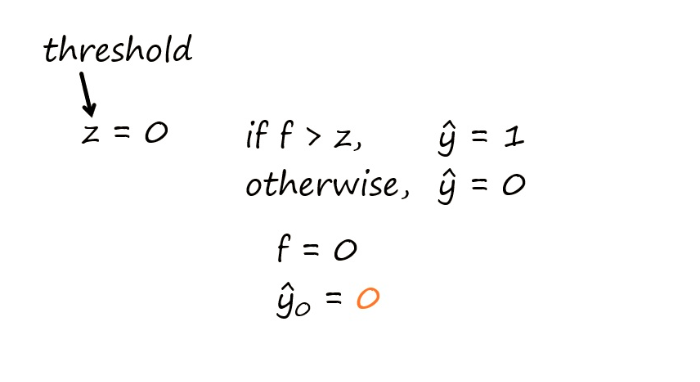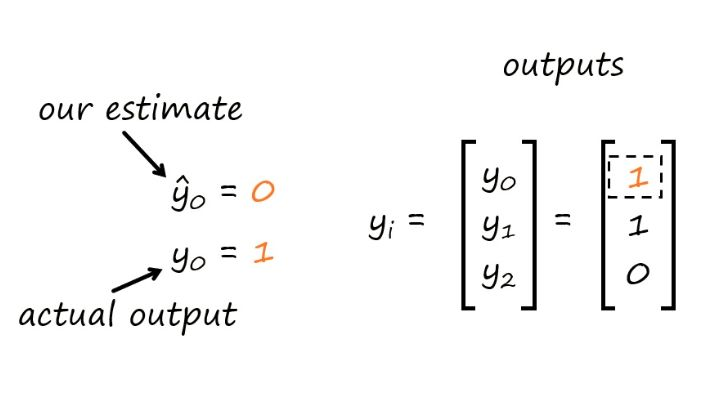4 更新权重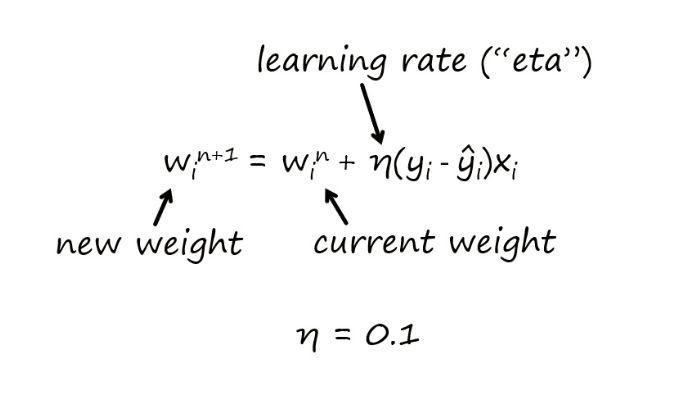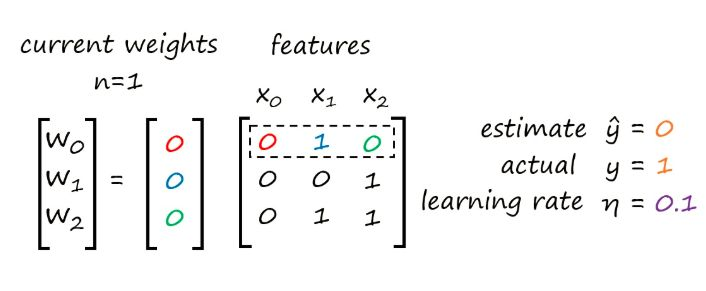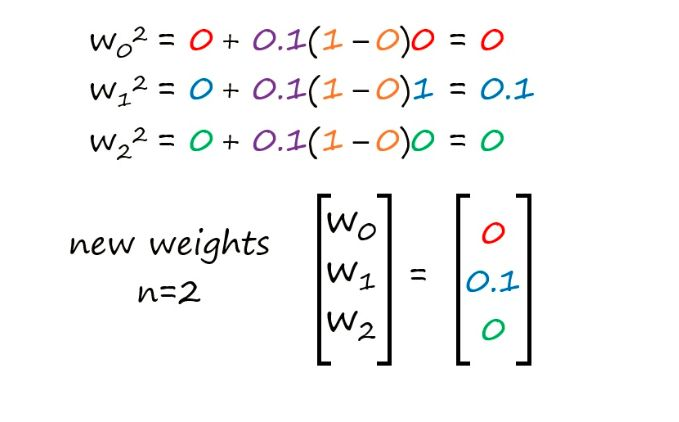5 重复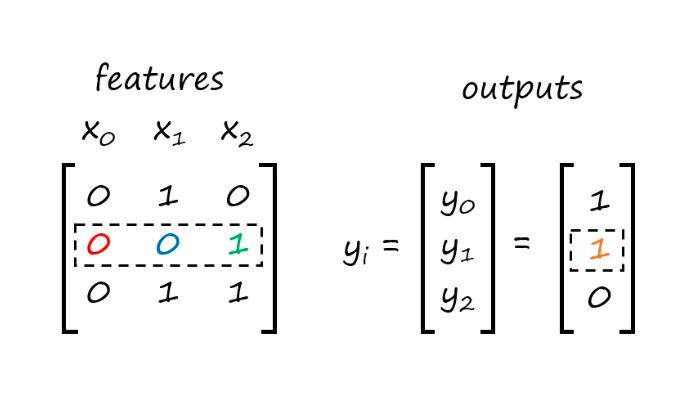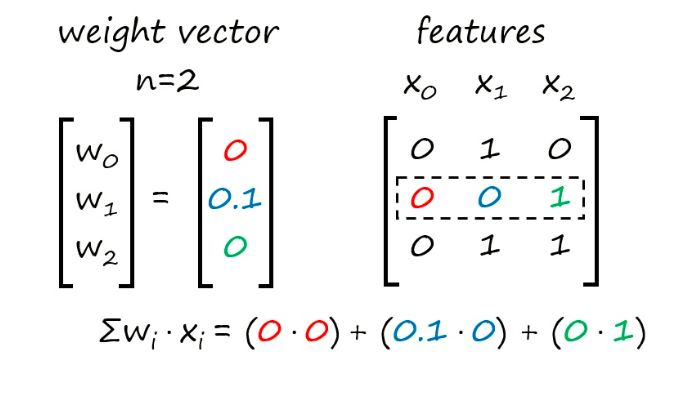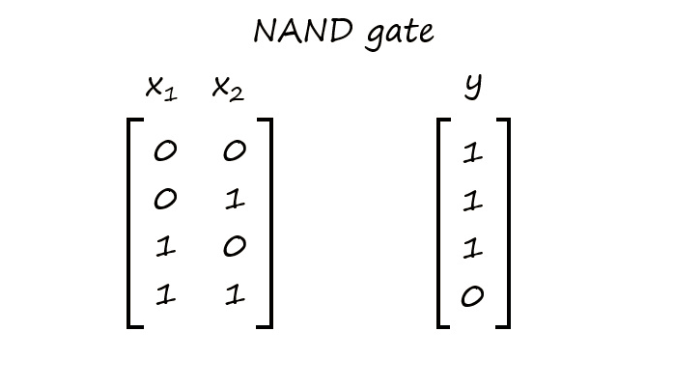1# Importing libraries

2# NAND Gate

3# Note:x0 is a dummy variable for the bias term

4# x0 x1 x2

5x = [[1., 0., 0.],

6[1., 0., 1.],

7[1., 1., 0.],

8[1., 1., 1.]]

9

10y =[1.,

111.,

121.,

130.]

1 初始化权重

1# Initialize the weights

2importnumpy asnp

3w = np.zeros(len(x[ 0]))

1Out:

2[ 0. 0. 0.]

2 将权重乘以输入，然后求和

1# Dot Product

2f = np.dot(w, x)

3print f

3 与阈值比较

1# Activation Function

2z = 0.0

3iff > z:

4yhat = 1.

5else:

6yhat = 0.

7

8printyhat

4 更新权重

1eta = 0.1

2w[ 0] = w[ 0] + eta *(y - yhat)*x[ 0][ 0]

3w[ 1] = w[ 1] + eta *(y - yhat)*x[ 0][ 1]

4w[ 2] = w[ 2] + eta *(y - yhat)*x[ 0][ 2]

5

6print w

5 重复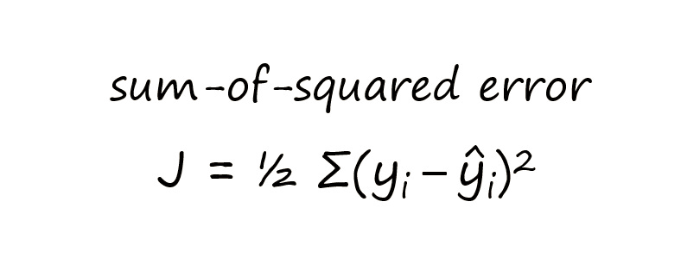1importnumpy asnp

2

3

4# Perceptron function

5defperceptron(x, y, z, eta, t):

6'''

7Input Parameters:

8x: data set of input features

9y: actual outputs

10z: activation function threshold

11eta: learning rate

12t: number of iterations

13'''

14

15# initializing the weights

16w = np.zeros(len(x[ 0]))

17n = 0

18

19# initializing additional parameters to compute sum-of-squared errors

20yhat_vec = np.ones(len(y)) # vector for predictions

21errors = np.ones(len(y)) # vector for errors (actual - predictions)

22J = [] # vector for the SSE cost function

23

24whilen < t: fori inxrange( 0, len(x)): # dot product f = np.dot(x[i], w) # activation function if f >= z:

25yhat = 1.

26else:

27yhat = 0.

28yhat_vec[i] = yhat

29

30# updating the weights

31forj inxrange( 0, len(w)):

32w[j] = w[j] + eta*(y[i]-yhat)*x[i][j]

33

34n += 1

35# computing the sum-of-squared errors

36fori inxrange( 0,len(y)):

37errors[i] = (y[i]-yhat_vec[i])** 2

38J.append( 0.5*np.sum(errors))

39

40returnw, J

1# x0 x1 x2

2x = [[1., 0., 0.],

3[1., 0., 1.],

4[1., 1., 0.],

5[1., 1., 1.]]

6

7y =[1.,

81.,

91.,

100.]

11

12z = 0.0

13eta = 0.1

14t = 50

15

16print "The weights are:"

17print perceptron(x, y, z, eta, t)

18

19print "The errors are:"

20print perceptron(x, y, z, eta, t)

• 导入数据
• 将数据分成训练集/测试集
• 训练我们的感知器
• 测试感知器
• 和scikit-learn的感知器相比

1 导入数据

1importpandas aspd

2importnumpy asnp

3importmatplotlib.pyplot asplt

4

5df = pd.read_csv( "dataset.csv")

6plt.scatter(df.values[:, 1], df.values[:, 2], c = df[ '3'], alpha= 0.8)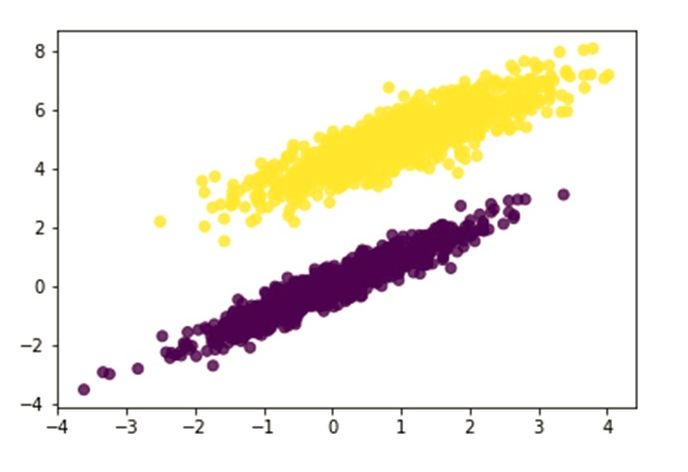2 将数据分成训练集/测试集

1df = df.values

2

3np.random.seed(5)

4np.random.shuffle(df)

1train = df[ 0: int( 0.7* len(df))]

2test = df[ int( 0.7* len(df)): int( len(df))]

1x_train= train[:, 0: 3]

2y_train= train[:, 3]

3

4x_test= test[:, 0: 3]

5y_test= test[:, 3]

3 训练我们的感知器

1defperceptron_train(x, y, z, eta, t):

2'''

3Input Parameters:

4x: data set of input features

5y: actual outputs

6z: activation function threshold

7eta: learning rate

8t: number of iterations

9'''

10

11# initializing the weights

12w = np.zeros(len(x[ 0]))

13n = 0

14

15# initializing additional parameters to compute sum-of-squared errors

16yhat_vec = np.ones(len(y)) # vector for predictions

17errors = np.ones(len(y)) # vector for errors (actual - predictions)

18J = [] # vector for the SSE cost function

19

20whilen < t: fori inxrange( 0, len(x)): # dot product f = np.dot(x[i], w) # activation function if f >= z:

21yhat = 1.

22else:

23yhat = 0.

24yhat_vec[i] = yhat

25

26# updating the weights

27forj inxrange( 0, len(w)):

28w[j] = w[j] + eta*(y[i]-yhat)*x[i][j]

29

30n += 1

31# computing the sum-of-squared errors

32fori inxrange( 0,len(y)):

33errors[i] = (y[i]-yhat_vec[i])** 2

34J.append( 0.5*np.sum(errors))

35

36returnw, J

37

38z = 0.0

39eta = 0.1

40t = 50

41

42perceptron_train(x_train, y_train, z, eta, t)

1w = perceptron_train(x_train, y_train, z, eta, t)

2J = perceptron_train(x_train, y_train, z, eta, t)

3

4print w

5print J

4测试我们的感知器

1fromsklearn.metrics importaccuracy_score

2

3w = perceptron_train(x_train, y_train, z, eta, t)[ 0]

4

5defperceptron_test(x, w, z, eta, t):

6y_pred = []

7fori inxrange( 0, len(x -1)):

8f = np.dot(x[i], w)

9

10# activation function

11iff > z:

12yhat = 1

13else:

14yhat = 0

15y_pred.append(yhat)

16returny_pred

17

18y_pred = perceptron_test(x_test, w, z, eta, t)

19

20print"The accuracy score is:"

21printaccuracy_score(y_test, y_pred)

5 和学过的感知器相比

1fromsklearn.linear_model importPerceptron

2

3# training the sklearn Perceptron

4clf = Perceptron(random_state= None, eta0= 0.1, shuffle= False, fit_intercept= False)

5clf.fit(x_train, y_train)

6y_predict = clf.predict(x_test)

scikit-learn模型中的权重与我们的相同。这意味着我们的模型工作正常，这是个好消息。

• 你会得到更深的理解，因为你正在教导别人你刚刚学到的东西。
• 你可以向潜在雇主展示它。